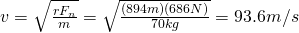# Rotational velocity and time related to artificial gravity

Last on our Physics questions for today:

Future space stations and spacecraft may create “artificial gravity” by rotating. Consider a cylindrical space station with a radius of 894 m that rotates about its central axis. The inside surface of the cylinder is the floor of the space station upon which the astronauts live and work. What period of rotation (in s) is necessary for a 70 kg astronaut to have the same weight as he has on Earth?

Now let’s identify what we have:

Astronaut Mass = 70 kg
Gravitational Acceleration (what we want it to be anyhow) = 9.8 m/s^2

The important thing to note is that we need to find the Normal Force being exerted on the astronaut as a result of the angular acceleration.  That force should be the equivalent of the Normal Force on Earth.  That pretty much tells us everything we need to get started.  Now, by this, we can figure the force intended by simple F = ma which gives us 686 N of force.  This is the Normal Force we want to attain.

Now, we need to find out at what velocity the cylinder needs to be spinning in order to obtain 686 N of force.  We can do this by using our angular velocity formula:Easy as pie right?  Now that we have the velocity, we need to find the total circumference, this will give us the distance that the cylinder has to rotate through in order to complete 1 revolution.Now that we have the total distance and we have velocity, our handy-dandy formula for distance, velocity and time can be used.And there you have it, it would take the space station 60 seconds to make one complete revolution in order to provide the same force on the astronaut as if on Earth.

As always, feel free to correct anything you see wrong with this.